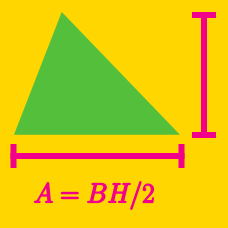Geometry

# Area of Triangles: Level 3 Challenges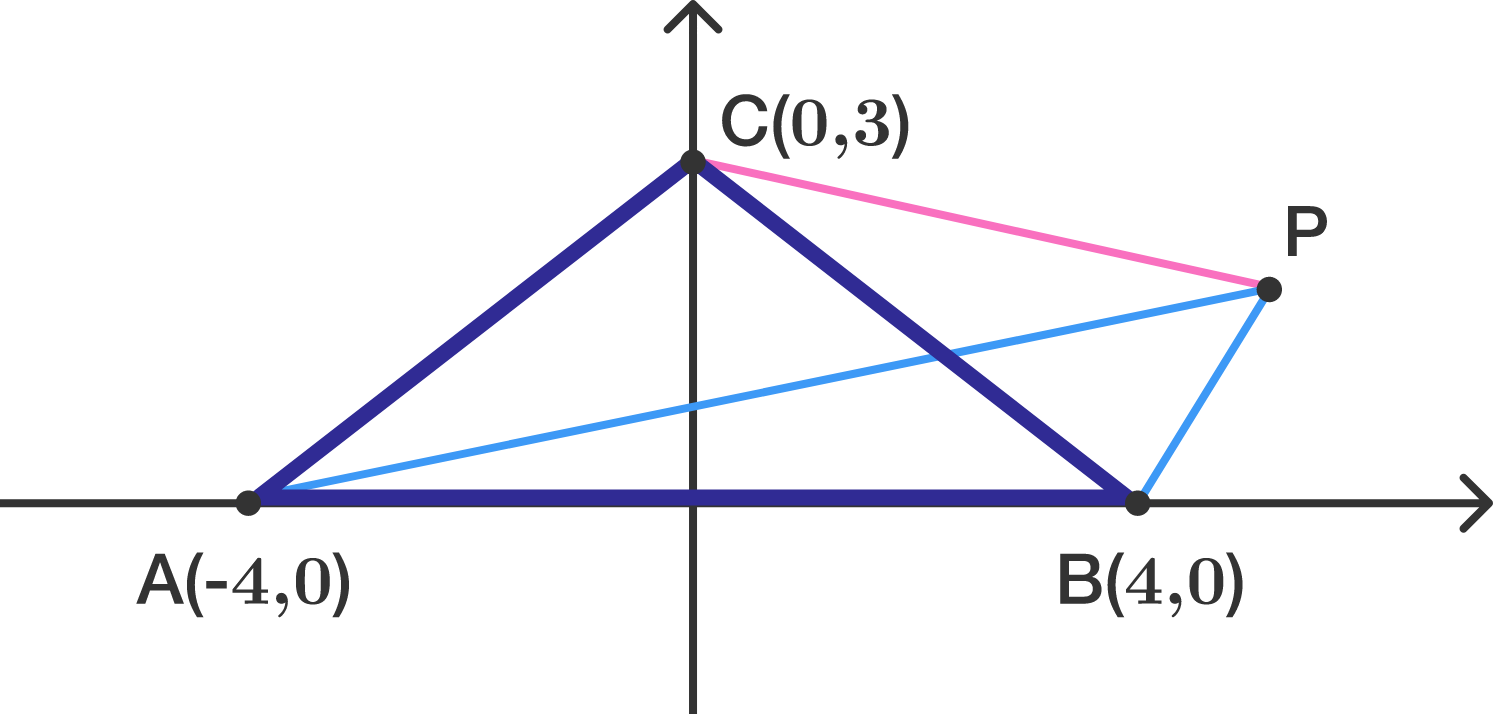Triangle $ABC$ has coodinates $A= (-4, 0)$, $B= (4 , 0)$, and $C= (0 , 3)$.

Let $P$ be the point in the first quadrant such that $\triangle ABP$ has half the area of $\triangle ABC$ but both triangles have the same perimeter.

What is the length of $CP?$ If your solution is in a form of $\sqrt{d}$, submit $d$ as the answer.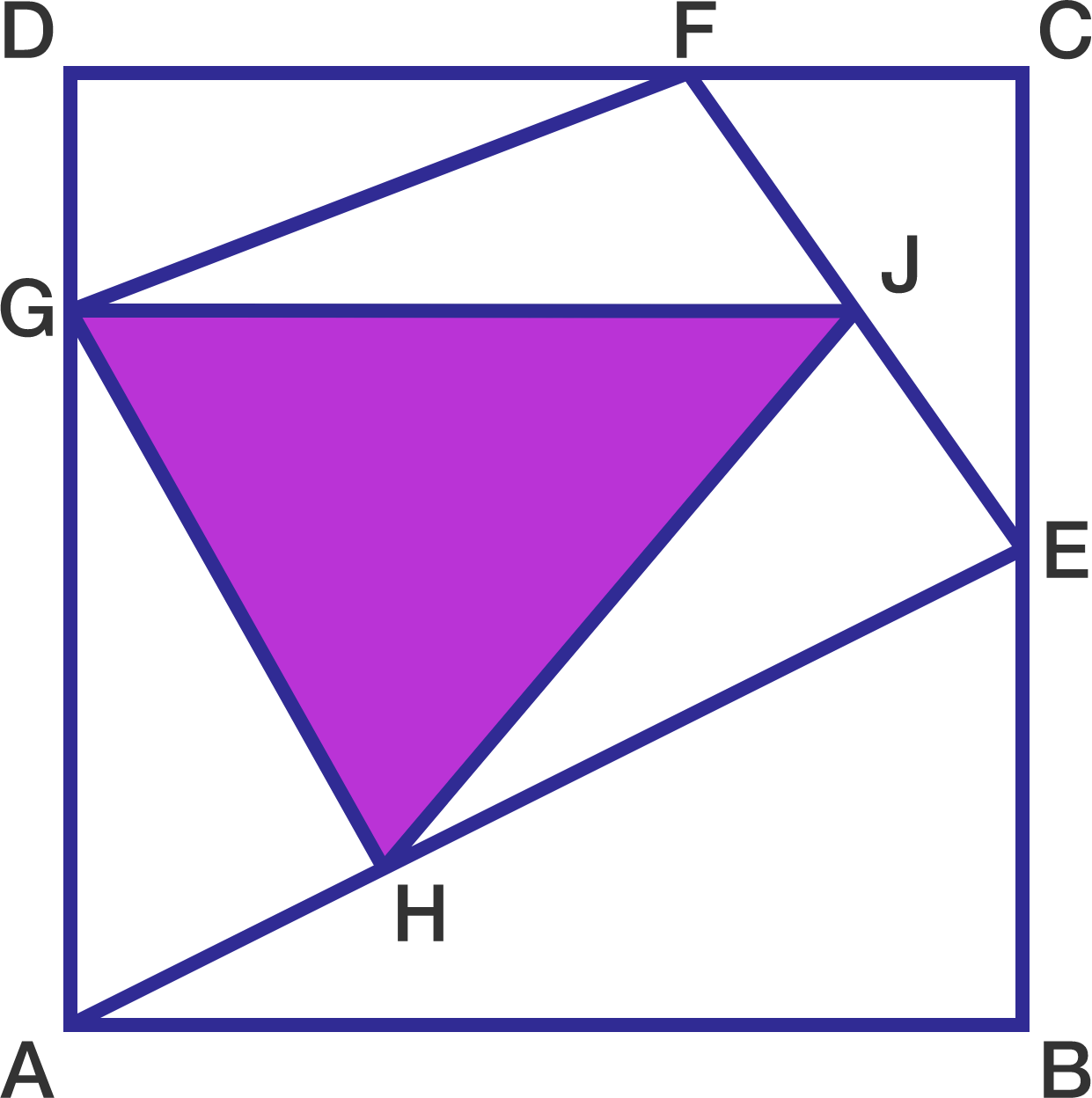Let $ABCD$ be a square of side length 12.

• $E$ is the midpoint of $CB$,
• $FC = \frac{1}{3} DC$,
• $GD = \frac{1}{4} DA$,
• $AH = \frac{1}{3} AE$,
• $J$ is the midpoint of $FE$.

What is the area of the purple triangle?

Which of the following triangles has a larger area:

• triangle A with side lengths $13, 13, 10$, or
• triangle B with side lengths $13, 13, 24\, ?$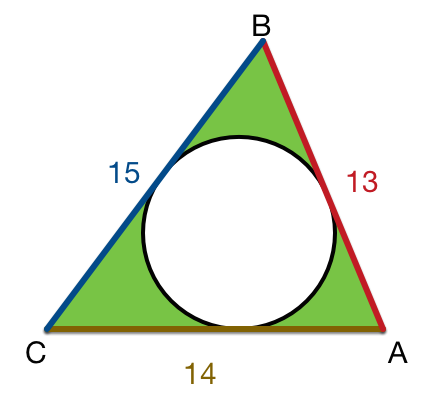In the figure above triangle $ABC$ with side-lengths $AC=14$, $AB=13$ and $BC=15.$ The incircle is drawn, which is tangential to all three sides. If the green shaded region is equal to $A$, find $\left\lfloor A\right\rfloor$.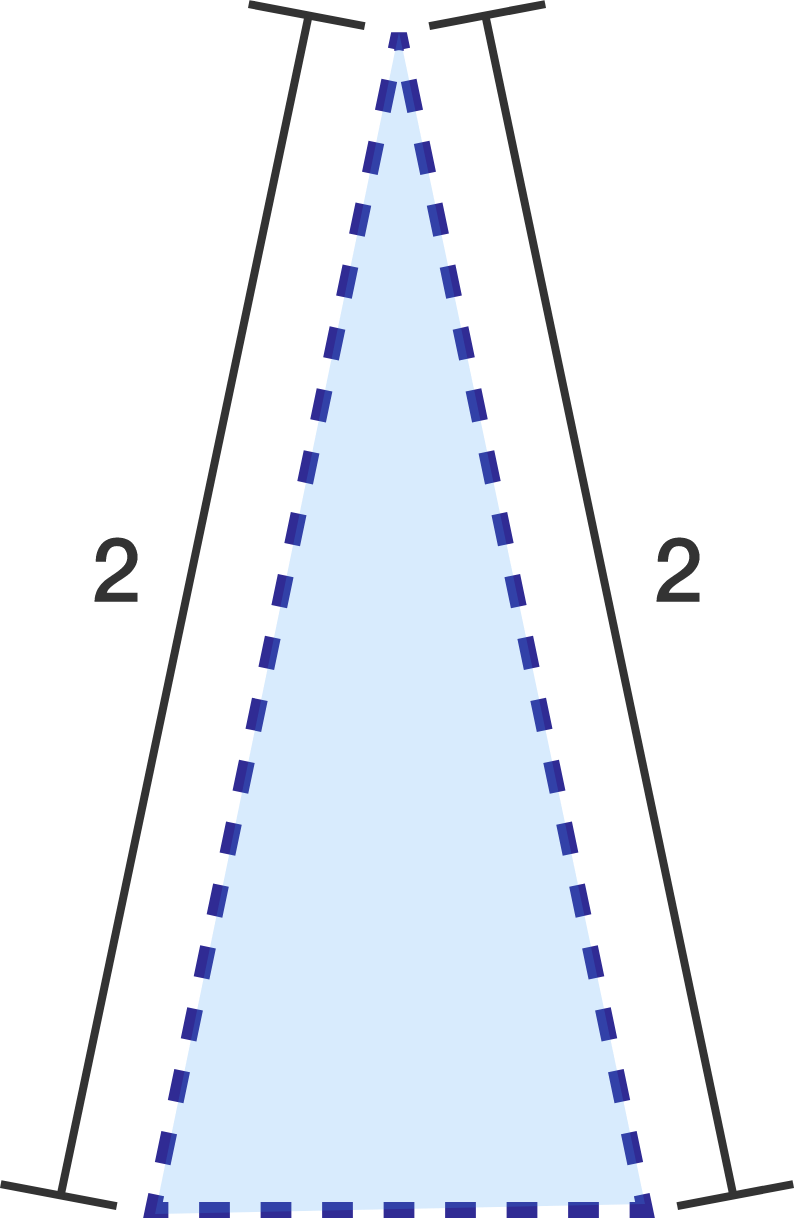What is the largest possible area of an isosceles triangle with two sides of length 2?

×